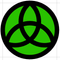Developer forum
cancel
Showing results for
Did you mean:

## Custom label to list out pipe invert levels?Contributor
I didn't try that and not sure if i'm right.

1. I guess you should read ConPosX, ConPosY, ConPosZ for each connection, which are here call "MEP_m_ConnectionsACL_4".

here was smth about labeling MEP elements
AC 22, 24 | Win 10
1 ACCEPTED SOLUTION
SolutionBooster
Coord Z of axis is storing in MEP_Position array.
```
dim _MEP_Position[] : _MEP_Position = 0 : dim1d1 = 1 : dim2d1 = 1
nd1 = request("Assoclp_parvalue", "MEP_Position", namindd1, typed1, flagd1, dim1d1, dim2d1, _MEP_Position)
dim MEP_Position[][] : MEP_Position = 0
n_rec = 1
for i=1 to dim1d1
for j=1 to dim2d1
MEP_Position = _MEP_Position[n_rec]
n_rec = n_rec + 1
next j
next i

MEP_StraightLength = 0
nd1 = request("Assoclp_parvalue", "MEP_StraightLength", namindd1, typed1, flagd1, dim1d1, dim2d1, MEP_StraightLength)

if vardim1(MEP_Position)>1 and vardim2(MEP_Position)=3 then
! --- x,y,z coordinates of 1st point ---
rx1 = MEP_Position
ry1 = MEP_Position
rz1 = MEP_Position
! --- x,y,z coordinates of 2nd point ---
rx2 = rx1+MEP_Position*MEP_StraightLength
ry2 = ry1+MEP_Position*MEP_StraightLength
rz2 = rz1+MEP_Position*MEP_StraightLength
endif

text2 0,0,rz1
text2 0,-0.3,rz2
```
https://github.com/kuvbur
4 REPLIES 4Virtuoso
Hotspots are rarely exposed parameters... You could probably pull the relevant info to work it out yourself though. No idea about MEP specific info. Given Smith's post, if your pipe is an actual MEP object, then the connection points are.

Ling.
AC18-23 AUS 7000
Self-taught, bend it till it breaks.
Win10 | E5620 x 2 | 24GB | K2200
SolutionBooster
Coord Z of axis is storing in MEP_Position array.
```
dim _MEP_Position[] : _MEP_Position = 0 : dim1d1 = 1 : dim2d1 = 1
nd1 = request("Assoclp_parvalue", "MEP_Position", namindd1, typed1, flagd1, dim1d1, dim2d1, _MEP_Position)
dim MEP_Position[][] : MEP_Position = 0
n_rec = 1
for i=1 to dim1d1
for j=1 to dim2d1
MEP_Position = _MEP_Position[n_rec]
n_rec = n_rec + 1
next j
next i

MEP_StraightLength = 0
nd1 = request("Assoclp_parvalue", "MEP_StraightLength", namindd1, typed1, flagd1, dim1d1, dim2d1, MEP_StraightLength)

if vardim1(MEP_Position)>1 and vardim2(MEP_Position)=3 then
! --- x,y,z coordinates of 1st point ---
rx1 = MEP_Position
ry1 = MEP_Position
rz1 = MEP_Position
! --- x,y,z coordinates of 2nd point ---
rx2 = rx1+MEP_Position*MEP_StraightLength
ry2 = ry1+MEP_Position*MEP_StraightLength
rz2 = rz1+MEP_Position*MEP_StraightLength
endif

text2 0,0,rz1
text2 0,-0.3,rz2
```
https://github.com/kuvburContributor
Hi,

current floor
```text2 0,0,rz1 + GLOB_ELEVATION
text2 0,-0.3,rz2 + GLOB_ELEVATION```
origin of the project
```text2 0,0,rz1 + SYMB_POS_Z
text2 0,-0.3,rz2 + SYMB_POS_Z```
Yves Houssier
Belgium
iMac - Mac Os 10,13Virtuoso
I see you are deducting the pipe diameter to get the invert. If the reported pipe coordinates are on the centreline then the z-value to obtain invert points will only be lowered by half the pipe diameter. It's a small thing but could be a big problem on site if your pipes are being installed 75mm lower than expected!
Apple iMac macOS Big Sur / AC24UKI (most recent builds)Latest solutions

Most liked posts